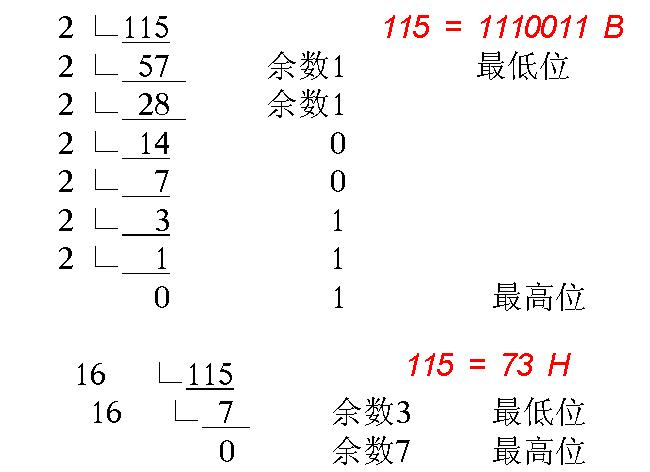# 写在前面

**本文共涉及两个Java程序（即两个类）

**

## 进制转换（数学方法）# 1、 转换为二进制算法解析

## 算法源码

// 将x转换为二进制方法
public int Two(int x) {
int t=0;
int a;
for(int i=1;x>0;i=i*10)
{
a=x%2;
x=x/2;
t=t+a*i;
}
return t;
}


# 2、 转换为八进制算法解析

## 算法源码

// 将x转换为八进制方法
public int Eight(int x) {
int t=0;
int a;
for(int i=1;x>0;i=i*10)
{
a=x%8;
x=x/8;
t=t+a*i;
}
return t;
}


# 3、 转换为十六进制算法解析

## 算法源码

// 将x转换为十六进制方法
public String Sixteen(int x) {
String t="";
int a;
for(;x>0;)
{
a=x%16;
switch(a)
{
case 10: t="A"+t; break;
case 11: t="B"+t; break;
case 12: t="C"+t; break;
case 13: t="D"+t; break;
case 14: t="E"+t; break;
case 15: t="F"+t; break;
default : t=a+t;
}
x=x/16;
}
return t;
}


（A:10 B:11 C:12 D:13 E:14 F:15）

# 工具类源码

// 工具类
public class BinaryTool {
public int Two(int x) {
int t=0;
int a;
for(int i=1;x>0;i=i*10)
{
a=x%2;
x=x/2;
t=t+a*i;
}
return t;
}
public int Eight(int x) {
int t=0;
int a;
for(int i=1;x>0;i=i*10)
{
a=x%8;
x=x/8;
t=t+a*i;
}
return t;
}
public String Sixteen(int x) {
String t="";
int a;
for(;x>0;)
{
a=x%16;
switch(a)
{
case 10: t="A"+t; break;
case 11: t="B"+t; break;
case 12: t="C"+t; break;
case 13: t="D"+t; break;
case 14: t="E"+t; break;
case 15: t="F"+t; break;
default : t=a+t;
}
x=x/16;
}
return t;
}

}


# 主程序源码

// 主程序
import java.util.Scanner;	//导入Scanner包
public class Shuru {
public static void main(String[] args) {
BinaryTool Tool = new BinaryTool();
Scanner print = new Scanner(System.in);
int x=print.nextInt();
System.out.println(Tool.Two(x));
System.out.println(Tool.Eight(x));
System.out.println(Tool.Sixteen(x));
}
}02-12370
03-091513
09-07788
03-121606
02-12279
03-13
05-306万+
05-057131
12-198219
06-061万+
10-301255
05-011109
03-051万+
08-26